# Cycle’s topological optimizations and the iterative decoding problem on general graphs final

13 de Oct de 2017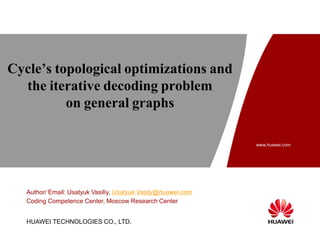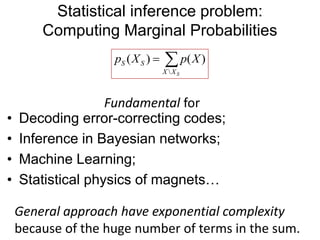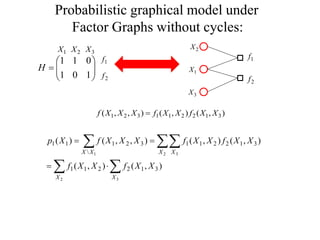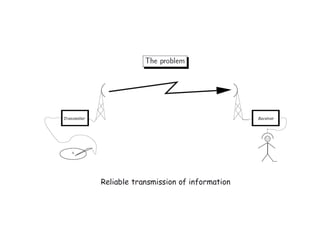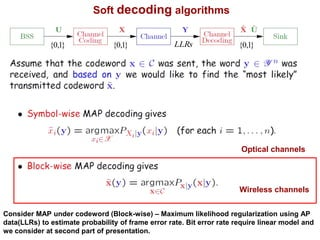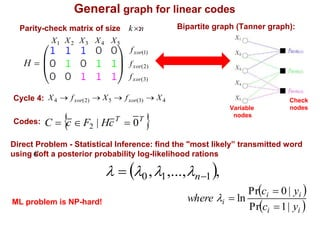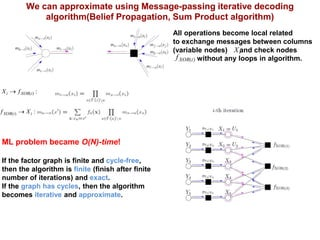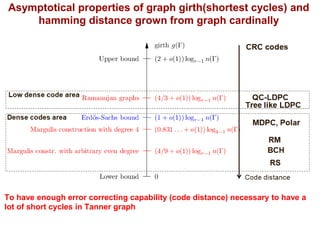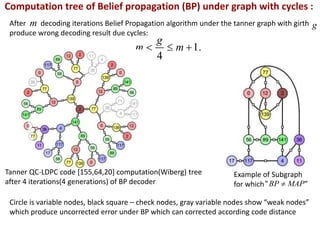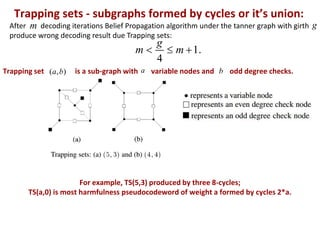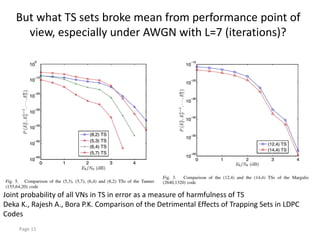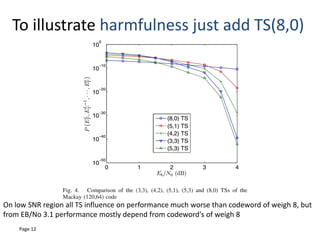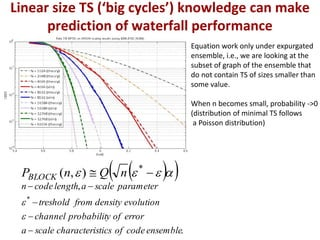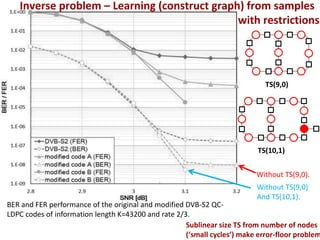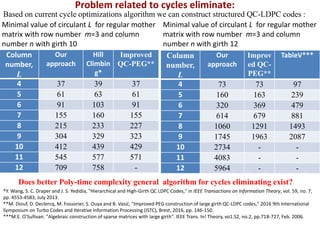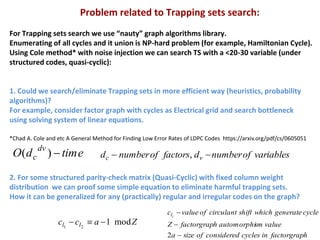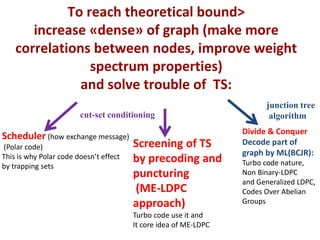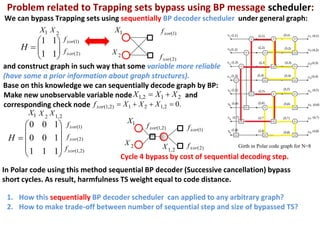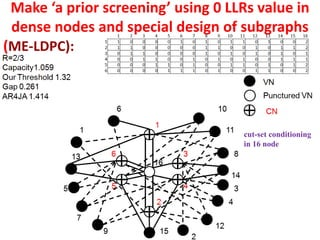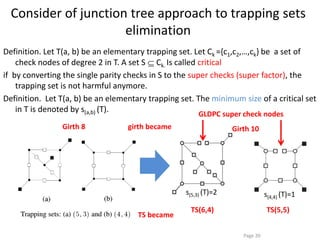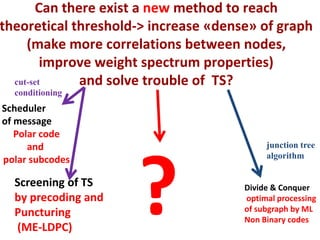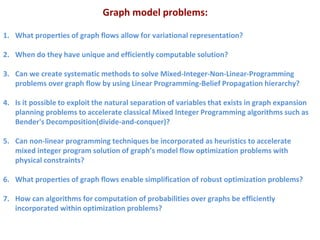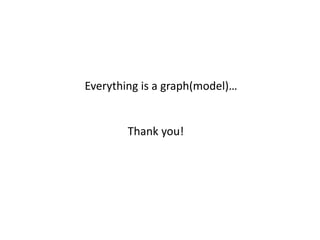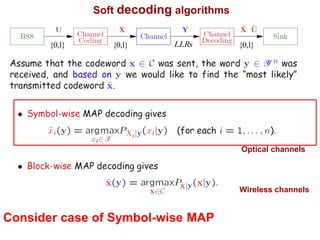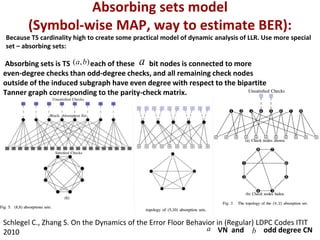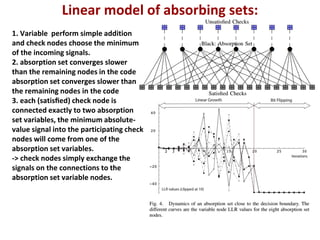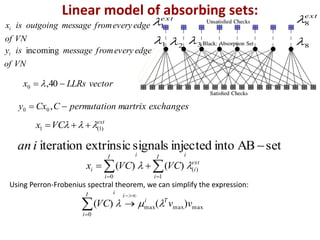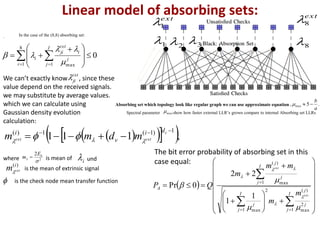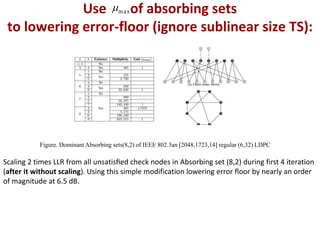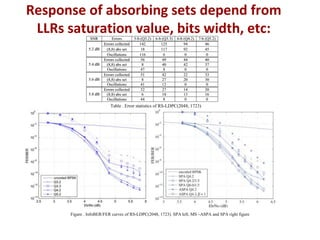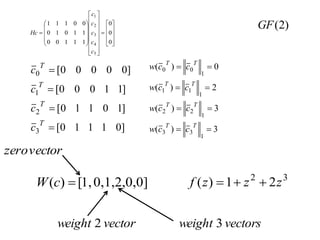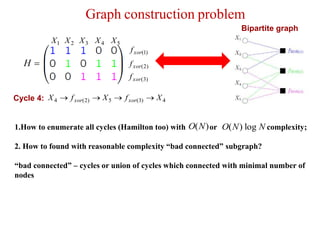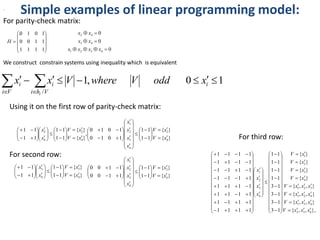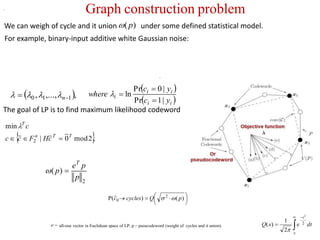1 de 34

### Cycle’s topological optimizations and the iterative decoding problem on general graphs final

• 1. www.huawei.com Security Level: HUAWEI TECHNOLOGIES CO., LTD. Cycle’s topological optimizations and the iterative decoding problem on general graphs Author/ Email: Usatyuk Vasiliy, Usatyuk.Vasily@huawei.com Coding Competence Center, Moscow Research Center
• 2. Statistical inference problem: Computing Marginal Probabilities • Decoding error-correcting codes; • Inference in Bayesian networks; • Machine Learning; • Statistical physics of magnets…  SXX SS XpXp )()( Fundamental for General approach have exponential complexity because of the huge number of terms in the sum.
• 3. Probabilistic graphical model under Factor Graphs without cycles:        101 011 H 321 XXX 2f 1f 1X 2X 3X 1f 2f ),(),(),,( 312211321 XXfXXfXXXf       2 3 2 31 ),(),( ),(),(),,()( 312211 312211 32111 X X X XXX XXfXXf XXfXXfXXXfXp
• 5. Soft decoding algorithms Wireless channels Optical channels Consider MAP under codeword (Block-wise) – Maximum likelihood regularization using AP data(LLRs) to estimate probability of frame error rate. Bit error rate require linear model and we consider at second part of presentation. LLRs }1,0{}1,0{}1,0{
• 6. General graph for linear codes Parity-check matrix of size : Codes:  TT cHFcC 0|2             11100 11010 00111 H Bipartite graph (Tanner graph): 54321 XXXXX Direct Problem - Statistical Inference: find the "most likely” transmitted word using soft a posterior probability log-likelihood rations    ii ii i yc yc where |1Pr |0Pr ln     ,,...,, 110  n ML problem is NP-hard! nk  4)3(5)2(4 XfXfX xorxor Cycle 4: )3(xorf )2(xorf )1(xorf c Variable nodes Check nodes
• 7. We can approximate using Message-passing iterative decoding algorithm(Belief Propagation, Sum Product algorithm) All operations become local related to exchange messages between columns (variable nodes) and check nodes without any loops in algorithm. iX )(iXORf ML problem became O(N)-time! If the factor graph is finite and cycle-free, then the algorithm is finite (finish after finite number of iterations) and exact. If the graph has cycles, then the algorithm becomes iterative and approximate. :)(iXORi fX  :)( iiXOR Xf 
• 8. Asymptotical properties of graph girth(shortest cycles) and hamming distance grown from graph cardinally To have enough error correcting capability (code distance) necessary to have a lot of short cycles in Tanner graph
• 9. After decoding iterations Belief Propagation algorithm under the tanner graph with girth produce wrong decoding result due cycles: .1 4  m g m Computation tree of Belief propagation (BP) under graph with cycles : m g Tanner QC-LDPC code [155,64,20] computation(Wiberg) tree after 4 iterations(4 generations) of BP decoder Circle is variable nodes, black square – check nodes, gray variable nodes show “weak nodes” which produce uncorrected error under BP which can corrected according code distance Example of Subgraph for which "" MAPBP 
• 10. After decoding iterations Belief Propagation algorithm under the tanner graph with girth produce wrong decoding result due Trapping sets: .1 4  m g m Trapping sets - subgraphs formed by cycles or it’s union: m g ),( baTrapping set is a sub-graph with a variable nodes and b odd degree checks. For example, TS(5,3) produced by three 8-cycles; TS(a,0) is most harmfulness pseudocodeword of weight a formed by cycles 2*a.
• 11. But what TS sets broke mean from performance point of view, especially under AWGN with L=7 (iterations)? Page 11 Joint probability of all VNs in TS in error as a measure of harmfulness of TS Deka K., Rajesh A., Bora P.K. Comparison of the Detrimental Effects of Trapping Sets in LDPC Codes
• 12. To illustrate harmfulness just add TS(8,0) Page 12 On low SNR region all TS influence on performance much worse than codeword of weigh 8, but from EB/No 3.1 performance mostly depend from codeword's of weigh 8
• 13. Linear size TS (‘big cycles’) knowledge can make prediction of waterfall performance     * ),( nQnPBLOCK . , * ensemblecodeofsticscharacteriscalea errorofyprobabilitchannel evolutiondensityfromtreshold parameterscalealengthcoden       Equation work only under expurgated ensemble, i.e., we are looking at the subset of graph of the ensemble that do not contain TS of sizes smaller than some value. When n becomes small, probability ->0 (distribution of minimal TS follows a Poisson distribution)
• 14. BER and FER performance of the original and modified DVB-S2 QC- LDPC codes of information length K=43200 and rate 2/3. TS(9,0) TS(10,1) Without TS(9,0). Without TS(9,0) And TS(10,1). Inverse problem – Learning (construct graph) from samples with restrictions Sublinear size TS from number of nodes (‘small cycles’) make error-floor problem
• 15. Problem related to cycles eliminate: Based on current cycle optimizations algorithm we can construct structured QC-LDPC codes : Could it be better cycle broke algorithm? *Y. Wang, S. C. Draper and J. S. Yedidia, "Hierarchical and High-Girth QC LDPC Codes," in IEEE Transactions on Information Theory, vol. 59, no. 7, pp. 4553-4583, July 2013. **M. Diouf, D. Declercq, M. Fossorier, S. Ouya and B. Vasić, "Improved PEG construction of large girth QC-LDPC codes," 2016 9th International Symposium on Turbo Codes and Iterative Information Processing (ISTC), Brest, 2016, pp. 146-150. ***M.E. O'Sullivan. "Algebraic construction of sparse matrices with large girth". IEEE Trans. In! Theory, vo1.S2, no.2, pp.718-727, Feb. 2006. Column number, L Our approach Hill Climbin g* Improved QC-PEG** 4 37 39 37 5 61 63 61 6 91 103 91 7 155 160 155 8 215 233 227 9 304 329 323 10 412 439 429 11 545 577 571 12 709 758 - Minimal value of circulant L for regular mother matrix with row number m=3 and column number n with girth 10 Column number, L Our approach Improv ed QC- PEG** TableV*** 4 73 73 97 5 160 163 239 6 320 369 479 7 614 679 881 8 1060 1291 1493 9 1745 1963 2087 10 2734 - - 11 4083 - - 12 5964 - - Minimal value of circulant L for regular mother matrix with row number m=3 and column number n with girth 12 Does better Poly-time complexity general algorithm for cycles eliminating exist?
• 16. Problem related to Trapping sets search: For Trapping sets search we use “nauty” graph algorithms library. Enumerating of all cycles and it union is NP-hard problem (for example, Hamiltonian Cycle). Using Cole method* with noise injection we can search TS with a <20-30 variable (under structured codes, quasi-cyclic): 1. Could we search/eliminate Trapping sets in more efficient way (heuristics, probability algorithms)? For example, consider factor graph with cycles as Electrical grid and search bottleneck using solving system of linear equations. 2. For some structured parity-check matrix (Quasi-Cyclic) with fixed column weight distribution we can proof some simple equation to eliminate harmful trapping sets. How it can be generalized for any (practically) regular and irregular codes on the graph? *Chad A. Cole and etc A General Method for Finding Low Error Rates of LDPC Codes https://arxiv.org/pdf/cs/0605051 timedO dv c )( variablesofnumberdfactorsofnumberd vc  , Zacc ll mod121  hfactorgrapincyclesconsideredofsizea valuesmautomorphihfactorgrapZ cyclegeneratewhichshiftcirculantofvaluec il    2
• 17. To reach theoretical bound> increase «dense» of graph (make more correlations between nodes, improve weight spectrum properties) and solve trouble of TS: Screening of TS by precoding and puncturing (ME-LDPC approach) Turbo code use it and It core idea of ME-LDPC Scheduler (how exchange message) (Polar code) This is why Polar code doesn’t effect by trapping sets Divide & Conquer Decode part of graph by ML(BCJR): Turbo code nature, Non Binary-LDPC and Generalized LDPC, Codes Over Abelian Groups cut-set conditioning junction tree algorithm
• 18. Problem related to Trapping sets bypass using BP message scheduler: We can bypass Trapping sets using sequentially BP decoder scheduler under general graph: In Polar code using this method sequential BP decoder (Successive cancellation) bypass short cycles. As result, harmfulness TS weight equal to code distance. Girth in Polar code graph for N=8 2X        11 11 H 1X )1(xorf )2(xorf 1X 2X )1(xorf )2(xorf and construct graph in such way that some variable more reliable (have some a prior information about graph structures). Base on this knowledge we can sequentially decode graph by BP: Make new unobservable variable node and corresponding check node 212,1 XXX  .02,121)2,1(  XXXfxor            111 100 100 H 1X 2X 2,1 X )1(xorf )2(xorf )2,1(xorf 1X 2X )1(xorf )2(xorf 2,1 X )2,1(xorf Cycle 4 bypass by cost of sequential decoding step. 1. How this sequentially BP decoder scheduler can applied to any arbitrary graph? 2. How to make trade-off between number of sequential step and size of bypassed TS?
• 19. Make ‘a prior screening’ using 0 LLRs value in dense nodes and special design of subgraphs (ME-LDPC): cut-set conditioning in 16 node
• 20. Consider of junction tree approach to trapping sets elimination Definition. Let T(a, b) be an elementary trapping set. Let Ck ={c1,c2,…,ck} be a set of check nodes of degree 2 in T. A set S Ck. Is called critical if by converting the single parity checks in S to the super checks (super factor), the trapping set is not harmful anymore. Definition. Let T(a, b) be an elementary trapping set. The minimum size of a critical set in T is denoted by s(a,b) (T). Page 20 s(5,3) (T)=2 s(4,4) (T)=1 GLDPC super check nodes TS became TS(6,4) TS(5,5) Girth 10Girth 8 girth became
• 21. Can there exist a new method to reach theoretical threshold-> increase «dense» of graph (make more correlations between nodes, improve weight spectrum properties) and solve trouble of TS? Screening of TS by precoding and Puncturing (ME-LDPC) Scheduler of message Polar code and polar subcodes Divide & Conquer optimal processing of subgraph by ML Non Binary codes ? junction tree algorithm cut-set conditioning
• 22. Graph model problems: 1. What properties of graph flows allow for variational representation? 2. When do they have unique and efficiently computable solution? 3. Can we create systematic methods to solve Mixed-Integer-Non-Linear-Programming problems over graph flow by using Linear Programming-Belief Propagation hierarchy? 4. Is it possible to exploit the natural separation of variables that exists in graph expansion planning problems to accelerate classical Mixed Integer Programming algorithms such as Bender's Decomposition(divide-and-conquer)? 5. Can non-linear programming techniques be incorporated as heuristics to accelerate mixed integer program solution of graph’s model flow optimization problems with physical constraints? 6. What properties of graph flows enable simplification of robust optimization problems? 7. How can algorithms for computation of probabilities over graphs be efficiently incorporated within optimization problems?
• 23. Everything is a graph(model)… Thank you!
• 24. Soft decoding algorithms Wireless channels Optical channels Consider case of Symbol-wise MAP LLRs }1,0{}1,0{}1,0{
• 25. Absorbing sets model (Symbol-wise MAP, way to estimate BER): Because TS cardinality high to create some practical model of dynamic analysis of LLR. Use more special set – absorbing sets: Absorbing sets is TS each of these bit nodes is connected to more even-degree checks than odd-degree checks, and all remaining check nodes outside of the induced subgraph have even degree with respect to the bipartite Tanner graph corresponding to the parity-check matrix. Schlegel C., Zhang S. On the Dynamics of the Error Floor Behavior in (Regular) LDPC Codes ITIT 2010 a VN and b odd degree CN ),( ba a
• 26. Linear model of absorbing sets: 1. Variable perform simple addition and check nodes choose the minimum of the incoming signals. 2. absorption set converges slower than the remaining nodes in the code absorption set converges slower than the remaining nodes in the code 3. each (satisﬁed) check node is connected exactly to two absorption set variables, the minimum absolute- value signal into the participating check nodes will come from one of the absorption set variables. -> check nodes simply exchange the signals on the connections to the absorption set variable nodes.
• 27. Linear model of absorbing sets: VNof edgeeveryfrommessageoutgoingisxi VNof edgeeveryfrommessageisyi incoming vectorLLRsx  40,0  exchangesmartrixnpermutatioCCxy  ,00 1 2 3 8 ext 1 ext 8 ext VCx )1(1   setABintoinjectedsignalsextrinsiciteration ian ext i iI i iI i i VCVCx )( 10 )()(     maxmaxmax 0 )()( vvVC Ti i iI i     Using Perron-Frobenius spectral theorem, we can simplify the expression:
• 28. Linear model of absorbing sets: 1 2 3 8 ext 1 ext 8                                   I j j j I j j I j j j A ext ext m m mm m QP 1 2 max )(2 1 max 1 max )( 1 1 22 0Pr        .5, max a b  max Absorbing set which topology look like regular graph we can use approximate equation Spectral parameter show how faster external LLR’s grown compare to internal Absorbing set LLRs In the case of the (8,8) absorbing set: 0 8 1 1 max              i I j j i ext ji i    . We can’t exactly know , since these value depend on the received signals. we may substitute by average values. which we can calculate using Gaussian density evolution calculation: ext ji     ,111 1)1(1)(   c extext di v i mdmm   The bit error probability of absorbing set in this case equal:where 2 2  bE m  is mean of i und )(i extm  is the check node mean transfer function is the mean of extrinsic signal
• 29. Use of absorbing sets to lowering error-floor (ignore sublinear size TS): max Scaling 2 times LLR from all unsatisﬁed check nodes in Absorbing set (8,2) during first 4 iteration (after it without scaling). Using this simple modification lowering error floor by nearly an order of magnitude at 6.5 dB. Figure. Dominant Absorbing sets(8,2) of IEEE 802.3an [2048,1723,14] regular (6,32) LDPC
• 30. Response of absorbing sets depend from LLRs saturation value, bits width, etc: Table . Error statistics of RS-LDPC(2048, 1723) Figure . InfoBER/FER curves of RS-LDPC(2048, 1723). SPA left, MS –ASPA and SPA right figure
• 32. Graph construction problem Bipartite graph 4)3(5)2(4 XfXfX xorxor Cycle 4:            11100 11010 00111 H 54321 XXXXX )3(xorf )2(xorf )1(xorf 1.How to enumerate all cycles (Hamilton too) with or complexity; 2. How to found with reasonable complexity “bad connected” subgraph? “bad connected” – cycles or union of cycles which connected with minimal number of nodes )(NO NNO log)(
• 33. Simple examples of linear programming model:. For parity-check matrix:            1111 1100 1010 H 0 0 0 4321 43 42    xxxx xx xx We construct constrain systems using inequality which is equivalent 10,1 /    i Vhi i Vi i xoddVwhereVxx i Using it on the first row of parity-check matrix: }{ }{ 11 11 1010 1010 , }{ }{ 11 11 11 11 4 2 4 3 2 1 4 2 4 2 xV xV x x x x xV xV x x                                                                For second row: }{ }{ 11 11 11 11 4 3 4 3 xV xV x x                           }{ }{ 11 11 1100 1100 4 3 4 3 2 1 xV xV x x x x                                      , . For third row: },,,{ },,{ },,{ },,{ }{ }{ }{ }{ 13 13 13 13 11 11 11 11 1111 1111 1111 1111 1111 1111 1111 1111 432 431 421 321 4 3 2 1 4 3 2 1 xxxV xxxV xxxV xxxV xV xV xV xV x x x x                                                                                               
• 34. Graph construction problem .        )()( 2 0 pQcyclesc  e all-one vector in Euclidean space of LP, p – pseucodeword (weight of cycles and it union). The goal of LP is to find maximum likelihood codeword  .2mod0| min 2 TTn T cHFcc c   . . 2 )( p pe p T      x t dtexQ 2 2 2 1 )(  We can weigh of cycle and it union under some defined statistical model.)(p    ii ii i yc yc where |1Pr |0Pr ln    ,,...,, 110  n For example, binary-input additive white Gaussian noise: